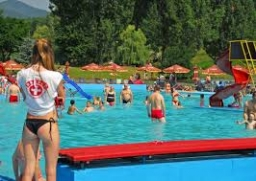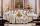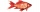# Water level

What is the area of the water level of the pool, if after filling 25 m3 of water level by 10 cm?
a) 25 m2
b) 250 m2
c) 2500 dm2
d) 25,000 cm2

Result

x = (Correct answer is: b)#### Solution:We would be pleased if you find an error in the word problem, spelling mistakes, or inaccuracies and send it to us. Thank you!Tips to related online calculators
Do you want to convert area units?
Do you want to convert length units?
Do you know the volume and unit volume, and want to convert volume units?

#### You need to know the following knowledge to solve this word math problem:

We encourage you to watch this tutorial video on this math problem:

## Next similar math problems:

• Here isHere is a data set (n=117) that has been sorted. 10.4 12.2 14.3 15.3 17.1 17.8 18 18.6 19.1 19.9 19.9 20.3 20.6 20.7 20.7 21.2 21.3 22 22.1 22.3 22.8 23 23 23.1 23.5 24.1 24.1 24.4 24.5 24.8 24.9 25.4 25.4 25.5 25.7 25.9 26 26.1 26.2 26.7 26.8 27.5 27.6 2
• Four prismsQuestion No. 1: The prism has the dimensions a = 2.5 cm, b = 100 mm, c = 12 cm. What is its volume? a) 3000 cm2 b) 300 cm2 c) 3000 cm3 d) 300 cm3 Question No.2: The base of the prism is a rhombus with a side length of 30 cm and a height of 27 cm. The heig
• Water levelHow high is the water in the swimming pool with dimensions of 37m in length and 15m in width, if an inlet valve is opened for 10 hours flowing 12 liters of water per second?
• Fire tank1428 hl of water is filled in a block-shaped fire tank with the edges of the base 12 m and 7 m. Calculate the content of water-wetted areas.
• RainfallHow many liters of water did fell in a 32m long and 8m wide garden, if 8mm of rain fell?
• Annual rainfallThe average annual rainfall is 686 mm. How many liters will fall on the 1-hectare field?
• The potThe pot is in 1/3 filled with water. Bottom of the pot has an area of ​​329 cm2. How many centimeters rises water level in the pot after add 1.2 liters of water?
• Circular poolThe base of the pool is a circle with a radius r = 10 m, excluding a circular segment that determines the chord length 10 meters. The pool depth is h = 2m. How many hectoliters of water can fit into the pool?
• PoolMr. Peter build a pool shape of a four-sided prism with rhombus base in the garden. Base edge length is 8 m, distance of the opposite walls of the pool is 7 m. Estimated depth is 144 cm. How many hectoliters of water consume Mr. Peter to fill the pool?
• RainfallAnnual rainfall in our country are an average of 797 mm. How many m3 of water rains on average per hectare?
• Sand pathHow many m3 of sand is needed to fill the 1.5m wide path around a rectangular flowerbed of 8m and 14m if the sand layer is 6cm high?
• The tankThe tank has 1320 liters of water. The tank has the shape of a prism, its base is an rectangle with sides a = 0,6 m and b = 1,5 m. How high does the water level reach in the tank?
• TrenchThe trench is a four-sided prism. The cross section has a trapezoidal shape with basements of 4m and 6m, the length of the trench is 30m. What is the depth of the trench if we dig 60,000 l of soil.
• Children poolThe bottom of the children's pool is a regular hexagon with a = 60 cm side. The distance of opposing sides is 104 cm, the height of the pool is 45 cm. A) How many liters of water can fit into the pool? B) The pool is made of a double layer of plastic film
• Iron poleWhat is the mass of pole with the shape of a regular quadrilateral prism with a length of 1 m and a cross-sectional side length of a = 4.5 cm make from iron with density ρ = 7800 kg/m³?
• AllanAllan keeps tropical fish. His aquarium is 4 feet long, 1 foot wide, and 2 feet tall. Each fish needs at least 0.5ft³ of water. What is the maximum number of fish that he can keep in the aquarium? Please show your solution. Please
• Surface and volume od cuboidContent area of the square base of cuboid is Sp = 36 cm2 and its height 80 mm. Determine its surface area and volume.